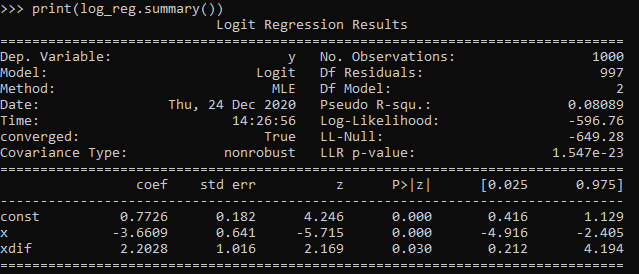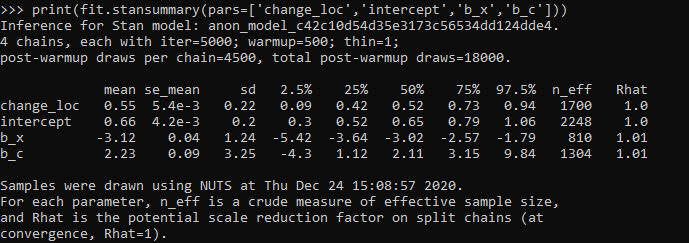# A changepoint logistic model in pystan

So the other day I showed how to use the mcp library in R to estimate a changepoint model with an unknown changepoint location. I was able to get a similar model to work in pystan, although it ends up being slower in practice than the mcp library (which uses JAGS under the hood). It also limits the changepoints to a specific grid of values. So offhand there isn’t a specific reason to prefer this approach to the R mcp library, but I post here to show my work. Also I illustrate that with this particular model, using 1000 simulated observations.

To be clear what this model is, instead of the many time series examples floating around about changepoints (like the one in the Stan guide), we have a model with a particular continuous independent variable x, and we are predicting the probability of something based on that x variable. It is not that different, but many of those time series examples the universe to check for changepoints is obvious, only the observed time series locations. But here we have a continuous input (distance a crime event is from a CCTV camera), but we can only check a finite number of locations. It ends up being closer in spirit to this recent post by Keith Goldfield.

So in some quick and dirty text math, here `c` is the changepoint location and `l` is the logit function:

``````l(Prob[y]) = intercept + b1*x; if x <= c
l(Prob[y]) = intercept + b1*x + b2*(x - c); if x > c``````

This model can be expanded however you want – such as other covariates that do not change with the changepoint. But for this simple simulation I am just looking at the one running variable x and the binary outcome y.

# Python Code

So first, I load up the libraries I will be using, then I simulate some data. Here the changepoint is located at 0.42 for the x variable, and in the `ylogit` line you can see the underlying logistic regression equation.

``````#################################
# Libraries I am using
import pystan
import numpy as np
import pandas as pd
import statsmodels.api as sm
#################################

#################################
# Creating simulated data
np.random.seed(10)
total_cases = 1000 #30000
x = np.random.uniform(size=total_cases) #[total_cases,1]
change = 0.42
xdif = (x - change)*(x > change)
ylogit = 1.1 + -4.3*x + 2.4*xdif
yprob = 1/(1 + np.exp(-ylogit))
ybin = np.random.binomial(1,yprob)
#################################``````

When testing out these models, one mistake I made was thinking offhand that 1,000 observations should be plenty. (Easier to run more draws with a smaller dataset.) When I had smaller effect sizes, the logistic coefficients could be pretty badly biased. So I started as a check estimating the logistic model inputting the correct changepoint location. Those biased estimates are pretty much the best case scenario you could hope for in the subsequent MCMC models. So here is an example fitting a logit model inputting the correct location for the changepoint.

``````#################################
#Statsmodel code to get
#The coefficient estimates
#And standard errors for the sims
con = *len(x)
xcomb = pd.DataFrame(zip(con,list(x),list(xdif)),columns=['const','x','xdif'])
log_reg = sm.Logit(ybin, xcomb).fit()
print(log_reg.summary())
#################################``````So you can see that my coefficient estimates and the frequentist standard errors are pretty large even with 1,000 samples. So I shouldn’t expect my later MCMC model to have any smaller credible intervals than above.

So here is the Stan model. I am using pystan here, but of course it would be the same text file if you wanted to fit the model using R. (Just compiles C++ code under the hood.) Of only real note is that I show how to use the softmax function to estimate the actual mean location of the changepoint. Note that that mean summary though only makes sense if you make your grid of changepoint locations regular and fairly fine. (So if you said a changepoint could be at 0.1, 0.36, and 0.87, taking a weighted mean of those three locations doesn’t make sense.)

``````#################################
#Stan model
changepoint_stan = """
data {
int<lower=1> N;
vector[N] x;
int<lower=0,upper=1> y[N];
int<lower=1> Samp_Points;
vector[Samp_Points] change;
}
transformed data {
real log_unif;
log_unif = -log(Samp_Points);
}
parameters {
real intercept;
real b_x;
real b_c;
}
transformed parameters {
vector[Samp_Points] lp;
real before;
real after;
lp = rep_vector(log_unif, Samp_Points);
for (c in 1:Samp_Points){
for (n in 1:N){
before = intercept + b_x*x[n];
after = before + b_c*(x[n] - change[c]);
lp[c] = lp[c] + bernoulli_logit_lpmf(y[n] | x[n] < change[c] ? before : after );
}
}
}
model {
intercept ~ normal(0.0, 10.0);
b_x ~ normal(0.0, 10.0);
b_c ~ normal(0.0, 10.0);
target += log_sum_exp(lp);
}
generated quantities {
vector[Samp_Points] prob_point;
real change_loc;
prob_point = softmax(lp);
change_loc = sum( prob_point .* change );
}
"""
#################################``````

And finally I show how to prepare the data for pystan (as a dictionary), compile the model, and then draw a ton of samples. I generate a regular grid of 0.01 intervals from 0.03 to 0.97 (can’t have a changepoint outside of the x data locations, which I drew as a random uniform 0,l). Note the more typical default of 1000 tended to not converge, the effective number of samples is quite small for that many. So 5k to 10k samples in my experiments tended to converge. Note that this is not real fast either, took about 40 minutes on my machine (the Stan guesstimates for time were always pretty good ballpark figures).

``````#################################
# Prepping data and fitting the model

stan_dat = {'N': ybin.shape}
stan_dat['change'] = np.linspace(0.03,0.97,95) #[0.1, 0.2, 0.3, 0.4, 0.5, 0.6, 0.7, 0.8, 0.9]
stan_dat['Samp_Points'] = len(stan_dat['change'])
stan_dat['x'] = x
stan_dat['y'] = ybin

sm = pystan.StanModel(model_code=changepoint_stan)
#My examples needed more like 10,000 iterations
#effective sample size very low, took about 40 minutes on my machine
fit = sm.sampling(data=stan_dat, iter=5000,
warmup=500, chains=4, verbose=True)
#Prints some results at the terminal!
print(fit.stansummary(pars=['change_loc','intercept','b_x','b_c']))
#################################``````So you can see the results – the credible intervals for the intercept and regression coefficient before the change point are not bad, just slightly larger than the logistic model. The credible interval for the changepoint location and the changepoint effect different are quite uncertain though. The changepoint location covers almost the whole interval I examined. It may be better to plot the individual probabilities, like Goldfield did in his post, as opposed to summarized a mean location for the distribution (which is discrete in the end based on your grid of locations you look at).

So that at least gives a partial warning that you need quite big data samples to effectively identify the changepoint location, at least for this Stan model as I have shown. I haven’t run it on my 26k actual data sample, as it will end up taking my computer around 30 hours to crunch out 10k draws with 4 chains. Next up I rather see if I can get a similar model working in pyro, as my GPU on my personal machine I think will be faster than the C++ code here. (There are probably smarter ways to vectorize the Stan model as well.)